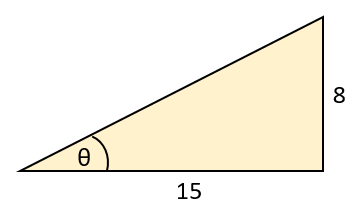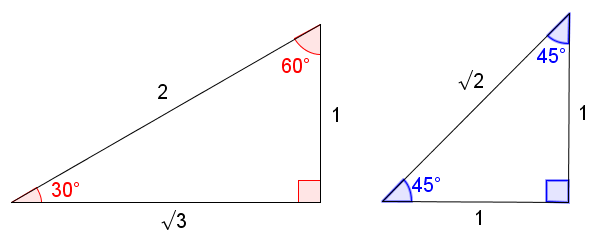# If Then Trigonometry

## Finding the exact values of sine, cosine and tangent of angles if given a different trig ratio.

##### MenuIf ThenCTR 1CTR 2CTR 3CTR 4CTR 5ExamHelpMore

Solve these "If Then" questions without using a calculator but giving exact answers in their simplest form. Use the / symbol to show a fraction and the root button to insert the square root sign if required.

 If $$\sin \theta = \frac{3}{5}$$then find $$\cos \theta$$If $$\tan \theta = \frac{3}{4}$$then find $$\sin \theta$$If $$\cos \theta = \frac{12}{13}$$then find $$\tan \theta$$If $$13\cos \theta = 5$$then find $$\sin \theta$$If $$3\tan \theta = 4$$then find $$\cos \theta$$If $$5\sin \theta = 4$$then find $$\tan \theta$$If $$2\cos \theta = 1$$then find $$\tan \theta$$If $$\tan \theta = 1$$then find $$\sin \theta$$If $$2\sin \theta = \sqrt{3}$$then find $$\cos \theta$$Check

## Instructions

Try your best to answer the questions above. Type your answers into the boxes provided leaving no spaces. As you work through the exercise regularly click the "check" button. If you have any wrong answers, do your best to do corrections but if there is anything you don't understand, please ask your teacher for help.

When you have got all of the questions correct you may want to print out this page and paste it into your exercise book. If you keep your work in an ePortfolio you could take a screen shot of your answers and paste that into your Maths file.

## Transum.org

This web site contains over a thousand free mathematical activities for teachers and pupils. Click here to go to the main page which links to all of the resources available.## More Activities:

Mathematicians are not the people who find Maths easy; they are the people who enjoy how mystifying, puzzling and hard it is. Are you a mathematician?

Comment recorded on the s /Indice 'Starter of the Day' page by Busolla, Australia:

"Thank you very much for providing these resources for free for teachers and students. It has been engaging for the students - all trying to reach their highest level and competing with their peers while also learning. Thank you very much!"

Comment recorded on the 6 May 'Starter of the Day' page by Natalie, London:

"I am thankful for providing such wonderful starters. They are of immence help and the students enjoy them very much. These starters have saved my time and have made my lessons enjoyable."

Each month a newsletter is published containing details of the new additions to the Transum website and a new puzzle of the month.

The newsletter is then duplicated as a podcast which is available on the major delivery networks. You can listen to the podcast while you are commuting, exercising or relaxing.

Transum breaking news is available on Twitter @Transum and if that's not enough there is also a Transum Facebook page.

##### Featured ActivityThe Transum Newsletter for June 2023 has just been published. Click on the image above to read about the latest developments on this site and try to solve the puzzle of the month. You can read the newsletter online or listen to it by downloading the podcast.

There are answers to this exercise but they are available in this space to teachers, tutors and parents who have logged in to their Transum subscription on this computer.

A Transum subscription unlocks the answers to the online exercises, quizzes and puzzles. It also provides the teacher with access to quality external links on each of the Transum Topic pages and the facility to add to the collection themselves.

Subscribers can manage class lists, lesson plans and assessment data in the Class Admin application and have access to reports of the Transum Trophies earned by class members.

Subscribe

## Go Maths

Learning and understanding Mathematics, at every level, requires learner engagement. Mathematics is not a spectator sport. Sometimes traditional teaching fails to actively involve students. One way to address the problem is through the use of interactive activities and this web site provides many of those. The Go Maths page is an alphabetical list of free activities designed for students in Secondary/High school.

## Maths Map

Are you looking for something specific? An exercise to supplement the topic you are studying at school at the moment perhaps. Navigate using our Maths Map to find exercises, puzzles and Maths lesson starters grouped by topic.

## Teachers

If you found this activity useful don't forget to record it in your scheme of work or learning management system. The short URL, ready to be copied and pasted, is as follows:

Alternatively, if you use Google Classroom, all you have to do is click on the green icon below in order to add this activity to one of your classes.

It may be worth remembering that if Transum.org should go offline for whatever reason, there are mirror sites at Transum.com and Transum.info that contain most of the resources that are available here on Transum.org.

When planning to use technology in your lesson always have a plan B!

Do you have any comments? It is always useful to receive feedback and helps make this free resource even more useful for those learning Mathematics anywhere in the world. Click here to enter your comments.For All:

Scan the QR code below to visit the online version of this activity.https://Transum.org/go/?Num=896

## Description of Levels

CloseSurds - Make sure you understand what surds are before starting the levels below.

Common Trig Ratios Level 1 - Find exact trig values for special angles up to and including ninety degrees

Common Trig Ratios Level 2 - Find the indicated lengths by solving trigonometric questions with exact solutions

Common Trig Ratios Level 3 - Mixed questions on exact trig values of special angles up to and including ninety degrees

Common Trig Ratios Level 4 - Find exact trig values for angles between ninety and three hundred and sixty degrees

Common Trig Ratios Level 5 - Solving trigonometric equations with given domains

Exam Style Questions - A collection of problems in the style of GCSE or IB/A-level exam paper questions (worked solutions are available for Transum subscribers).

More Trigonometry including visual aids, investigations and self-marking exercises.

Answers to this exercise are available lower down this page when you are logged in to your Transum account. If you don’t yet have a Transum subscription one can be very quickly set up if you are a teacher, tutor or parent.

## Help

The questions in this exercise are designed to be solved by drawing a diagram of a right-angled triangle, choosing the lenghts of two of the sides using the given ratio then use Pythagoras' theorem to figure out the length of the third side. The required trig ratio can then be found from the diagram.

For example, if $$\tan \theta = \frac{8}{15}$$ then find $$\sin \theta$$

Firstly sketch a righ-angled triangle containing the angle $$\theta$$, opposite 8 and adjacent 15.The length of the hypotenuse can be calculated using pythagoras' Theorem to be $$\sqrt{8^2 + 15^2} = 17$$.

Finally $$\sin \theta$$ can be calculated as the opposite over the hypotenuse which is $$\frac{8}{17}$$.

## Common Trigonometric Ratios Video